﻿ “7.19”中国中东部地区暴雨过程诊断分析

# “7.19”中国中东部地区暴雨过程诊断分析A Diagnostic Analysis of “7.19” Rainstorm over Central and Eastern China

Abstract: Using NCEP/NCAR daily 4 times the FNL global reanalysis and CMORPH hourly precipitation data, the large-area rainstorm process occurring on July 19, 2016 are the diagnosed and analyzed. The results show that the southwest vortex is formed greatly influenced by the stable two troughs and two ridges over high latitudes, the Western Pacific subtropical high and the short wave trough over Qinghai-Tibet plateau. The stable of high latitude trough and Western Pacific subtropical high over middle and low latitudes are favorable for the eastward movement of southwest vortex and vortex over North China, the water vapor transport and the unstable energy accumulation, and finally create favorable conditions for the occurrence of rainstorm. The wet Q vector also has a good indicator effect on the next 6 h precipitation. In the areas with obvious upward movement in the middle and lower troposphere in the rainstorm area, unstable stratification is easy to be stimulated to generate strong convective activities, corresponding to strong wet Q convergence. Therefore, the wet Q convergence area is positively correlated with the precipitation intensity. The large positive center of Z-helicity area in the middle and lower troposphere has a good indication for the precipitation area in the next 6 hours. The distribution of the positive Z-helicity area in the horizontal direction is consistent with the main precipitation area. The center of positive Z-helicity area corresponds to strong convergence ascending motion, which is an effective physical quantity for rainstorm forecast.

1. 引言

2. 资料与方法

2.1. 资料

2.2. 方法

(1) 湿Q矢量

${Q}_{x}^{\ast }=\frac{1}{2}\left[f\left(\frac{\partial v}{\partial p}\frac{\partial u}{\partial x}-\frac{\partial u}{\partial p}\frac{\partial v}{\partial x}\right)-h\frac{\partial V}{\partial x}\cdot \nabla \text{θ}+\frac{\partial \left(hH\right)}{\partial x}\right]$ (1)

${Q}_{y}^{\ast }=\frac{1}{2}\left[f\left(\frac{\partial v}{\partial p}\frac{\partial u}{\partial y}-\frac{\partial u}{\partial p}\frac{\partial v}{\partial y}\right)-h\frac{\partial V}{\partial y}\cdot \nabla \text{θ}+\frac{\partial \left(hH\right)}{\partial y}\right]$ (2)

${Q}_{x}^{\ast }$${Q}_{y}^{\ast }$ 分别表示在x和y方向上的湿Q分量。在不考虑凝结潜热作用下，其本质与Hoskins等  定义的准地转湿Q一致。在考虑凝结潜热的作用下，作 $\frac{\partial }{\partial \text{x}}\left(2\right)+\frac{\partial }{\partial \text{y}}\left(3\right)$ 得湿Q矢量散度为强迫项的非地转ω方程：

${\nabla }^{2}\left(\sigma \omega \right)+{f}^{2}\frac{{\partial }^{2}\omega }{\partial {p}^{2}}=-2\nabla \cdot {Q}^{\ast }$ (3)

(2) 湿-Z螺旋度：

$F=w\zeta =w\left(\frac{\partial v}{\partial x}-\frac{\partial u}{\partial y}\right)=-\frac{\omega }{pg}\left(\frac{\partial v}{\partial x}-\frac{\partial u}{\partial y}\right)$ (4)

3. 研究结果

3.1. 环流形势与降水过程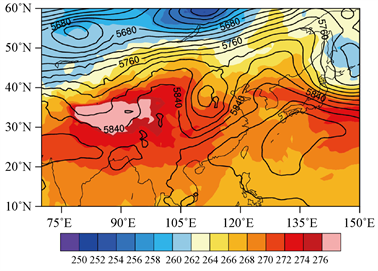(a)(b)

Figure 1. 500 hPa height field and temperature field at 12 hours on (a) 19 and (b) 20 July 2016 (colouring map, unit: ˚C)

3.2. 假相当位温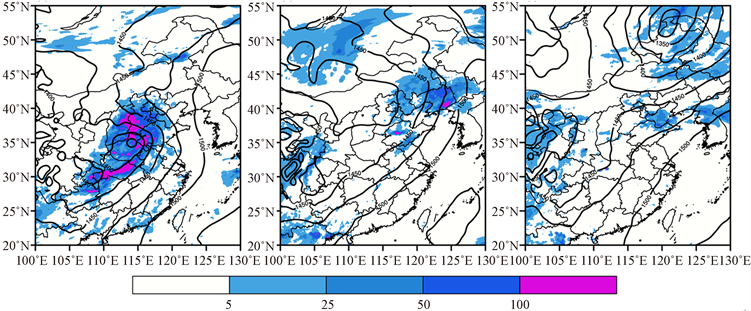(a) (b) (c)

Figure 2. Accumulated precipitation in 24 h (colouring map, unit: mm) and the geopotential height of 700 hPa at 12:00 on the corresponding day (contour line), (a) 18th, (b) 20th and (c) 22th on July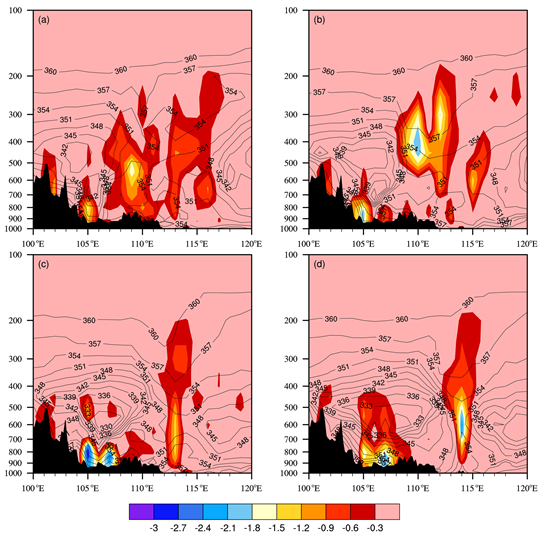Figure 3. Vertical velocity along 32˚N (colorimetric map, unit Pa/s) and ${\theta }_{se}$ (line, unit K), black shadows indicate terrain, (a) 00:00, (b) 06:00, (c) 12:00, (d) 18:00 on 19th, July

3.3. 湿Q矢量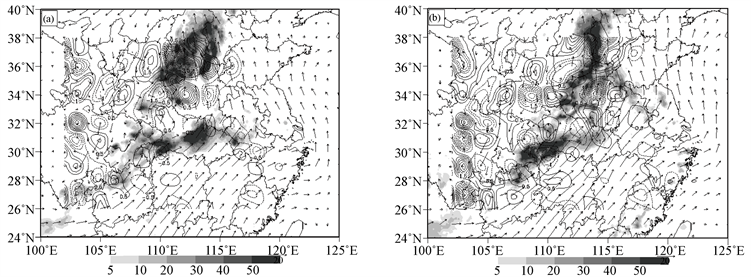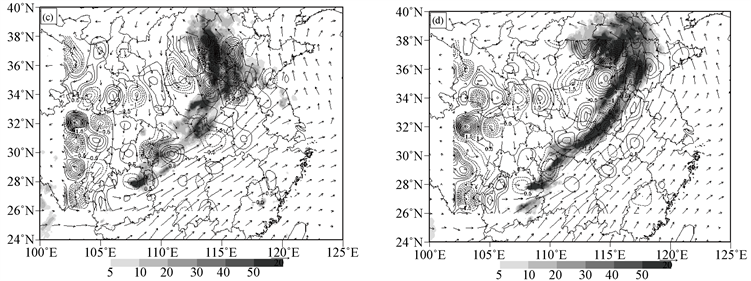Figure 4. The wet Q vector divergence (line, unit: 1 × 10−15 hPa−1∙s −3) and the accumulated precipitation in the future 6 h at this moment (sheading, unit: mm), (a) 00:00, (b) 06:00, (c) 12:00 and (d) 18:00 on 19th July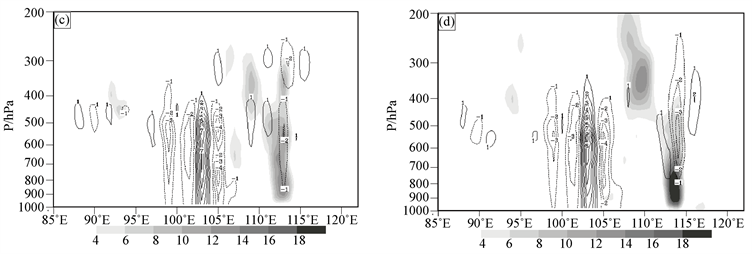Figure 5. Vorticity (shadow, unit: 1 × 10−5 s−1) and non-geodetic wetting Q divergence (contour line, unit: 1 × 10−15 hPa−1∙s−3) along the vortex center，(a) 00:00, (b) 06:00, (c) 12:00 and (d) 18:00 on 19th July

3.4. Z-螺旋度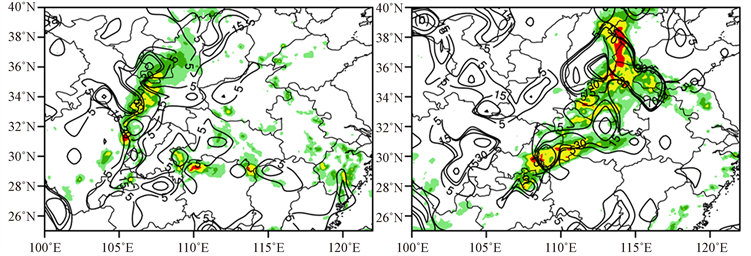(a) (b)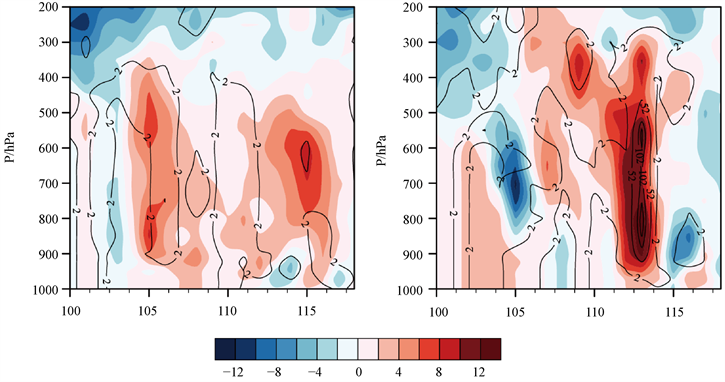(c) (d)

Figure 6. Z-helicity (unit: 10−6 m∙s−2) and 6 h precipitation (shaded area, unit: mm) horizontal distribution (a) at 12:00 on July 18 and (b) 12:00 on July 19. Vertical profiles of relative vorticity (shaded area, unit: 10−5 s−1) and Z-helicity (unit: 10−6 m∙s−1) along 32˚N at 12:00; (c) on July 18, 2016 and 12:00; (d) on July 19, 2016

4. 结论

(1) 受中高纬度稳定的两槽两脊结构的影响，低纬华南地区受西太平洋副热带高压的控制，以及青藏高原短波槽的影响，西南涡在四川东部生成。高纬度槽和中低纬度西太副高的稳定维持，西南涡和华北上空低涡的东移和水汽输送和不稳定能量的积蓄为暴雨的发生创造了有利条件。

(2) 湿Q矢量对未来6 h降水也有很好的指示作用，暴雨区对流层中低层上升运动明显的地方，易激发出不稳定层结，产生强对流活动，对应强的湿Q辐合，因此湿Q辐合区与降水强度呈正相关。

(3) 对流层中底层Z-螺旋度正值区大值中心也对未来6 h降水落区有较好的指示意义，在水平方向上，Z-螺旋度正值密集区分布和主要降水区仍然保持一致。Z-螺旋度正值区中心对应气流的辐合上升作用强，是暴雨预报的有效物理量。

 陶诗言, 等. 中国之暴雨[M]. 北京: 科学出版社, 1980: 196-199.

 刘红武, 李国平. 三十年西南低涡研究的回顾与展望[J]. 高原山地气象研究, 2008, 28(2): 68-72.

 赵思雄, 傅慎明. 2004年9月川渝大暴雨期间西南低涡结构及其环境场的分析[J]. 大气科学, 2007, 31(6): 1059-1075.

 史小康, 李耀东, 刘健文, 等. 华北一次暴雨过程的螺旋度分析[J]. 自然灾害学报, 2012, 21(4): 48-56.

 吴国雄, 刘还珠. 全型垂直涡度倾向方程和倾斜涡度发展[J]. 气象学报, 1999, 57(1): 1-14.

 朱禾, 邓北胜, 吴洪. 湿位涡守恒条件下西南涡的发展[J]. 气象学报, 2002, 60(3): 343-351.

 Hoskins, B.J., Draghici, I. and Davies, H.C. (1978) A New Look at the M-Equation. Quarterly Journal of the Royal Meteorological Society, 104, 31-38.
https://doi.org/10.1002/qj.49710443903

 杨晓霞, 沈桐立, 刘还珠, 等. 非地转湿Q矢量分解在暴雨分析中的应用[J]. 高原气象, 2006(3): 464-475.

 黄楚惠, 李国平. 基于螺旋度和非地转湿Q矢量的一次东移高原低涡强降水过程分析[J]. 高原气象, 2009, 28(2): 319-326.

 张雪琦, 白爱娟. “15•8”川东西南涡暴雨的Q矢量分析[J]. 高原山地气象研究, 2015, 35(3): 32-38.

 王璐思, 陈林琳, 张晓涵, 等. 四川盆地2013年夏季三次特大暴雨过程对比分析[J]. 高原山地气象研究, 2015, 35(1): 86-91.

 史小康, 李耀东, 刘健文, 等. 华北一次暴雨过程的螺旋度分析[J]. 自然灾害学报, 2012, 21(4): 48-56.

 张兴旺. 湿Q矢量表达式及其应用[J]. 气象, 1998(8): 4-8.

 Lilly, D.K. (1986) The Structure, Energetics, and Propagation of Rotating Convective Storms. Part II: Helicity and Storm Stabilization. Journal of the Atmospheric Sciences, 43, 126-140.
https://doi.org/10.1175/1520-0469(1986)043<0126:TSEAPO>2.0.CO;2

 李耀辉, 寿绍文. 旋转风螺旋度及其在暴雨演变过程中的作用[J]. 南京气象学院学报, 1999(1): 98-105.

Top